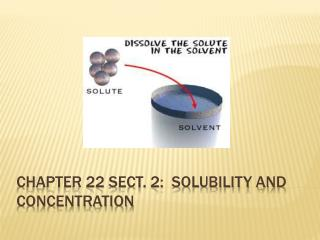# Chapter 22 Sect. 2: Solubility and Concentration - PowerPoint PPT PresentationDownload PresentationChapter 22 Sect. 2: Solubility and Concentration

Chapter 22 Sect. 2: Solubility and ConcentrationDownload Presentation## Chapter 22 Sect. 2: Solubility and Concentration

- - - - - - - - - - - - - - - - - - - - - - - - - - - E N D - - - - - - - - - - - - - - - - - - - - - - - - - - -
##### Presentation Transcript

1. Chapter 22 Sect. 2: Solubility and Concentration

2. What has to take place in order for a solute to be dissolved? • Solvent molecules surround the solute particles and pull the solute particles into solution, and the complex spreads out evenly throughout the solution Dissolving Review

3. What determines the amount of solute that is able to dissolve in a solvent? • Solubility: the maximum amount of solute that can be dissolved in a given amount of solvent at a given temperature • Solubility depends on the properties of the solute and solvent • Some solutes are more “soluble” in some solvents than others • This means that more of it can be dissolved in that particular solvent than in a different solvent, or more will dissolve in a solvent compared to another solute Solubility

4. The concentration of a solution refers to the amount of solute dissolvedin a solution • Concentrated: Solutions with a large amount of solute particles • Diluted: Solutions with a small amount of solute particles Concentrated vs. Diluted

5. To find the concentration of a solution: • Divide the mass of the solute by the volume of solvent • We will use units of grams of solute per 100 mL of solvent (g/100mL) • i.e. the concentration of a sample of salt water is 35.9 g/100 mL of water • You may need to set up a proportion to determine concentration Concentrations of Solutions =

6. 1. What is the concentration of a solution in grams/100 mL when 40 grams of solute dissolve in 200 mL of solution? 2. A solution contains 15 grams of solute in 30 mL of solution. What is the concentration of the solution in g/100 mL? 3. A solution has a concentration of 2 g/ 100 mL. If you have 600 mL of the solution, how many grams of solute do you have? Calculating Concentrations = x = 20 g Answer: 20 g/100mL = x = 50 Answer: 50 g/100mL = x = 12 Answer: 12 g

7. There are three other terms that can be used to describe the concentration of a solution based on the amount of solute that is dissolved • Saturated– a solution that contains all of the possible solute that it can hold at a given temperature (no more solute can dissolve) • Unsaturated– a solution that can dissolve more solute at a given temperature • Supersaturated– a solution that contains more solute than a saturated solution at the same temperature • Usually in order to produce a supersaturated solution, the solution needs to be heated which increases the solubility of most solids • Gases on the other hand are more soluble in liquids when the temperature is decreased Types of Solutions

8. Our classroom has 27 desks… • If there are < 27students: unsaturated classroom – more students can still fit in the class • If there are 27students: saturated classroom – can not fit anymore students • If there are > 27students: supersaturated classroom– adding more causes students to be displaced, or fall into aisles (precipitation) • Increase temperature, students up and moving around the room, more energy, more students can fit in the classroom Saturation analogy

9. A solubility curve is a graph showing the relationship between solubility and temperature (or sometimes pressure) • We can use solubility curves to figure out how much solute will dissolve in a particular solvent at a certain temperature • We can also use them to determine if a solution is saturated, unsaturated, or supersaturated • If above the curve: • If on the line of the curve: • If below the curve: Solubility curves • supersaturated • saturated • unsaturated

10. Use the solubility curve to the right to answer the following questions. What is the solubility of Ba(OH)2 in 100 mL of water at 80˚C? At about what temperature will 100g of water dissolve equal amounts of KNO3 and NaNO3? (Hint: 100g water = 100 mL water) How many grams of KNO3 are needed to produce a saturated solution at 40˚C? If 120g of NaNO3 are dissolved in 100g of water at 20˚C, is the solution saturated, unsaturated or super saturated? If 70g of KI are dissolved in 100g of water at 30˚C, is the solution saturated, unsaturated or super saturated? Solubility curves Practice ~95g/100 mL water ~73˚C ~65g Supersaturated (above the solubility line) Unsaturated (below the solubility line)